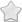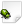CLOSED  Crash report #1488  -  [5FBBA383] Thread::entryPoint() basic_string.h:2290
Posted Jul 27, 2020 - updated Aug 20, 2021   Shortlink: http://arx.vg/1488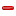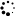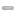0 Votes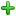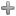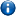This issue is a duplicate of issue #1094 - "[F4AE7D16] Thread::entryPoint() Thread.cpp:144"This issue has been closed with status "Not a bug" and resolution "DUPLICATE".
Issue details
• Type of issue
Crash report
• Status
 Not a bug
• Assigned to
Not assigned to anyone
• Type of bug
Not triaged
• Likelihood
Not triaged
• Effect
Not triaged
• Posted by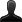CrashBot
• Owned by
Not owned by anyone
• Estimated time
Not estimated
• Category
Not determined
• Resolution
DUPLICATE
• Priority
Not determined
• Reproducability
Not determined
• Severity
Not determined
• Targetted for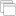Not determined
• OS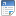Linux
• ArchitectureOther
• Fixed inNot determined
Issue description
Illegal storage access: address not mapped to object

``` Instruction address: 0x103c2c
Memory accessed: 0xffffffff81000020
Stack pointer: 0xa2bfee38
Frame pointer: 0xa2bfef7c
```

Callstack:
``` arx!0x10d1ac
??!0xb6767130
arx!0x103c2c
```

GDB stack trace:
``` [New LWP 19652]
[New LWP 19653]
[New LWP 19654]
[New LWP 19655]
0xb6ebb510 in memcmp () from /usr/lib/arm-linux-gnueabihf/libarmmem-v7l.so
Id   Target Id                                   Frame
* 1    Thread 0xb6ef8010 (LWP 19649) "arx"         0xb6ebb510 in memcmp () from /usr/lib/arm-linux-gnueabihf/libarmmem-v7l.so
2    Thread 0xb4421440 (LWP 19652) "threaded-ml" __GI___poll (timeout=449, nfds=3, fds=0x264a4b8) at ../sysdeps/unix/sysv/linux/poll.c:29
3    Thread 0xa3c20440 (LWP 19653) "arx"         futex_wait_cancelable (private=0, expected=0, futex_word=0x2642478) at ../sysdeps/unix/sysv/linux/futex-internal.h:88
4    Thread 0xa2fff440 (LWP 19654) "arx"         0xb6e7e088 in futex_abstimed_wait_cancelable (private=0, abstime=0x0, expected=1, futex_word=0x2647314) at ../sysdeps/unix/sysv/linux/futex-internal.h:205
5    Thread 0xa2bff440 (LWP 19655) "arx"         __GI___nanosleep (remaining=0x0, requested_time=0xa2bfea98) at ../sysdeps/unix/sysv/linux/nanosleep.c:28

#0  __GI___nanosleep (remaining=0x0, requested_time=0xa2bfea98) at ../sysdeps/unix/sysv/linux/nanosleep.c:28
_a1 = -1564480872
_nr = 162
_a1tmp = -1564480872
_a2tmp = 0
_a2 = 0
_sys_result = <optimized out>
sc_cancel_oldtype = 0
sc_ret = <optimized out>
sc_ret = <optimized out>
sc_ret = <optimized out>
_sys_result = <optimized out>
_a1 = <optimized out>
_nr = <optimized out>
_a2tmp = <optimized out>
_a1tmp = <optimized out>
_a2 = <optimized out>
sc_cancel_oldtype = <optimized out>
_sys_result = <optimized out>
_a1 = <optimized out>
_nr = <optimized out>
_a2tmp = <optimized out>
_a1tmp = <optimized out>
_a2 = <optimized out>
#1  __GI___nanosleep (requested_time=0xa2bfea98, remaining=0x0) at ../sysdeps/unix/sysv/linux/nanosleep.c:25
sc_ret = <optimized out>
_sys_result = <optimized out>
_a1 = <optimized out>
_nr = <optimized out>
_a2tmp = <optimized out>
_a1tmp = <optimized out>
_a2 = <optimized out>
sc_cancel_oldtype = <optimized out>
_sys_result = <optimized out>
_a1 = <optimized out>
_nr = <optimized out>
_a2tmp = <optimized out>
_a1tmp = <optimized out>
_a2 = <optimized out>
#2  0x0010d20c in CrashHandlerPOSIX::handleCrash (context=<optimized out>, info=0xa2bfeac0, signal=<optimized out>, this=0x2398460) at /home/pi/sc/arx/src/platform/crashhandler/CrashHandlerPOSIX.cpp:501
t = {tv_sec = 0, tv_nsec = 100000}
processor = 19656
args = {0x0, 0x186a0 <__static_initialization_and_destruction_0(int, int) [clone .constprop.416] 36264> "\002", 0x0}
processor = <optimized out>
args = <optimized out>
it = <optimized out>
siginfo = <optimized out>
ctx = <optimized out>
t = <optimized out>
#3  signalHandler (signal=<optimized out>, info=0xa2bfeac0, context=<optimized out>) at /home/pi/sc/arx/src/platform/crashhandler/CrashHandlerPOSIX.cpp:85
No locals.
#4  <signal handler called>
No locals.
#5  0x00103c2c in Thread::entryPoint (param=0x81000010) at /usr/include/c  /8/bits/basic_string.h:2290
ret = <optimized out>
start = <optimized out>
pd = 0xa2bff440
unwind_buf = {cancel_jmp_buf = {{jmp_buf = {1593130831, 1252889951, -1225782936, -1564478400, -1225816880, 338, -1090565526, -1564478400, 0, -1564479620, 0 <repeats 54 times>}, mask_was_saved = 0}}, priv = {pad = {0x0, 0x0, 0x0, 0x0}, data = {prev = 0x0, cleanup = 0x0, canceltype = 0}}}
not_first_call = <optimized out>
#7  0xb6811578 in ?? () at ../sysdeps/unix/sysv/linux/arm/clone.S:73 from /lib/arm-linux-gnueabihf/libc.so.6
No locals.
Backtrace stopped: previous frame identical to this frame (corrupt stack?)

#0  0xb6e7e088 in futex_abstimed_wait_cancelable (private=0, abstime=0x0, expected=1, futex_word=0x2647314) at ../sysdeps/unix/sysv/linux/futex-internal.h:205
_a2tmp = 393
_a2 = 393
_v2 = -1
_a3tmp = 1
_a3 = 1
_a1 = 40137492
_nr = 240
_a4tmp = 0
_a1tmp = 40137492
_a4 = 0
_v1 = 0
__ret = <optimized out>
oldtype = 0
err = <optimized out>
oldtype = <optimized out>
err = <optimized out>
__ret = <optimized out>
_a1 = <optimized out>
_nr = <optimized out>
_v2tmp = <optimized out>
_v1tmp = <optimized out>
_a4tmp = <optimized out>
_a3tmp = <optimized out>
_a2tmp = <optimized out>
_a1tmp = <optimized out>
_a2 = <optimized out>
_a3 = <optimized out>
_a4 = <optimized out>
_v1 = <optimized out>
_v2 = <optimized out>
#1  do_futex_wait (sem=sem@entry=0x2647314, abstime=0x0) at sem_waitcommon.c:115
err = <optimized out>
#2  0xb6e7e1f4 in __new_sem_wait_slow (sem=0x2647314, abstime=0x0) at sem_waitcommon.c:282
_buffer = {__routine = 0xb6e7e050 <__sem_wait_cleanup>, __arg = 0x2647314, __canceltype = 1868739072, __prev = 0x0}
err = 0
v = <optimized out>
#3  0xb6d0e7b0 in ?? () from /usr/lib/arm-linux-gnueabihf/libopenal.so.1
No symbol table info available.
Backtrace stopped: previous frame identical to this frame (corrupt stack?)

#0  futex_wait_cancelable (private=0, expected=0, futex_word=0x2642478) at ../sysdeps/unix/sysv/linux/futex-internal.h:88
_a1 = 40117368
_nr = 240
_a3tmp = 0
_a1tmp = 40117368
_a3 = 0
_a4tmp = 0
_a2tmp = 128
_a2 = 128
_a4 = 0
__ret = <optimized out>
oldtype = 0
err = <optimized out>
oldtype = <optimized out>
err = <optimized out>
__ret = <optimized out>
_a1 = <optimized out>
_nr = <optimized out>
_a4tmp = <optimized out>
_a3tmp = <optimized out>
_a2tmp = <optimized out>
_a1tmp = <optimized out>
_a2 = <optimized out>
_a3 = <optimized out>
_a4 = <optimized out>
spin = 0
buffer = {__routine = 0xb6e7ae28 <__condvar_cleanup_waiting>, __arg = 0xa3c1fd98, __canceltype = 0, __prev = 0x0}
cbuffer = {wseq = 60, cond = 0x2642450, mutex = 0xb4705188, private = 0}
__atg101_val = <optimized out>
rt = <optimized out>
__atg100_val = <optimized out>
err = <optimized out>
g = 0
flags = <optimized out>
g1_start = <optimized out>
signals = <optimized out>
result = 0
wseq = 257698037760
seq = 11745413666745630472
private = 0
maxspin = <optimized out>
err = <optimized out>
result = <optimized out>
wseq = <optimized out>
g = <optimized out>
seq = <optimized out>
flags = <optimized out>
private = <optimized out>
signals = <optimized out>
g1_start = <optimized out>
__atg6_oldval = <optimized out>
__atg6_memp = <optimized out>
__atg6_value = <optimized out>
__atg2_result = <optimized out>
__oldval = <optimized out>
__atg101_val = <optimized out>
__atg100_val = <optimized out>
spin = <optimized out>
buffer = <optimized out>
cbuffer = <optimized out>
__atg101_val = <optimized out>
__atg100_val = <optimized out>
__atg6_oldval = <optimized out>
__atg6_memp = <optimized out>
__atg6_value = <optimized out>
__atg2_result = <optimized out>
__oldval = <optimized out>
__atg101_val = <optimized out>
__atg100_val = <optimized out>
rt = <optimized out>
__atg101_val = <optimized out>
__atg100_val = <optimized out>
__atg102_expected = <optimized out>
__atg1_result = <optimized out>
__oldval = <optimized out>
s = <optimized out>
__atg100_val = <optimized out>
__atg102_expected = <optimized out>
__atg1_result = <optimized out>
__oldval = <optimized out>
No locals.
#3  0xb485b084 in pa_threaded_mainloop_wait () from /usr/lib/arm-linux-gnueabihf/libpulse.so.0
No symbol table info available.
#4  0xb6d06c68 in ?? () from /usr/lib/arm-linux-gnueabihf/libopenal.so.1
No symbol table info available.
Backtrace stopped: previous frame identical to this frame (corrupt stack?)

#0  __GI___poll (timeout=449, nfds=3, fds=0x264a4b8) at ../sysdeps/unix/sysv/linux/poll.c:29
_a1 = 40150200
_nr = 168
_a3tmp = 449
_a1tmp = 40150200
_a3 = 449
_a2tmp = 3
_a2 = 3
_sys_result = <optimized out>
sc_cancel_oldtype = 0
sc_ret = <optimized out>
sc_ret = <optimized out>
sc_ret = <optimized out>
_sys_result = <optimized out>
_a1 = <optimized out>
_nr = <optimized out>
_a3tmp = <optimized out>
_a2tmp = <optimized out>
_a1tmp = <optimized out>
_a2 = <optimized out>
_a3 = <optimized out>
sc_cancel_oldtype = <optimized out>
_sys_result = <optimized out>
_a1 = <optimized out>
_nr = <optimized out>
_a3tmp = <optimized out>
_a2tmp = <optimized out>
_a1tmp = <optimized out>
_a2 = <optimized out>
_a3 = <optimized out>
#1  __GI___poll (fds=0x264a4b8, nfds=3, timeout=449) at ../sysdeps/unix/sysv/linux/poll.c:26
sc_ret = <optimized out>
_sys_result = <optimized out>
_a1 = <optimized out>
_nr = <optimized out>
_a3tmp = <optimized out>
_a2tmp = <optimized out>
_a1tmp = <optimized out>
_a2 = <optimized out>
_a3 = <optimized out>
sc_cancel_oldtype = <optimized out>
_sys_result = <optimized out>
_a1 = <optimized out>
_nr = <optimized out>
_a3tmp = <optimized out>
_a2tmp = <optimized out>
_a1tmp = <optimized out>
_a2 = <optimized out>
_a3 = <optimized out>
#2  0xb485a968 in ?? () from /usr/lib/arm-linux-gnueabihf/libpulse.so.0
No symbol table info available.
Backtrace stopped: previous frame identical to this frame (corrupt stack?)

#0  0xb6ebb510 in memcmp () from /usr/lib/arm-linux-gnueabihf/libarmmem-v7l.so
No symbol table info available.
#1  0x000c8ddc in std::char_traits<char>::compare (__n=<optimized out>, __s2=<optimized out>, __s1=0x2acc211 "ok_for_shany 0\t\t// 1= player searching 2= shani free 3= shani dead\r\n set #need_dragon_egg 0\r\n set #need_form 0\r\n set #need_krahoz 0\r\n set #need_shield 0\t\t// alia asked for shield\r\n set #need_superweap"...) at /usr/include/c  /8/bits/char_traits.h:312
No locals.
#2  std::__cxx11::basic_string<char, std::char_traits<char>, std::allocator<char> >::find (__n=<optimized out>, __pos=<optimized out>, __s=<optimized out>, this=<optimized out>) at /usr/include/c  /8/bits/basic_string.tcc:1214
__elem0 = 111 'o'
__data = 0x2ac9358 "on game_ready {\r\n if (\247fighting_amb == 0) ambiance -v 0 ambient_fight\r\naccept\r\n}\r\n\r\non init {\r\nset #debug 0\r\nset \247cheat 0\r\n//timerf 0 2 herosay ^#player_life\r\n //set #crashtest 1\r\n set #shut_up 0\r\n lo"...
__last = 0xc8ddc <loadScript(EERIE_SCRIPT&, PakFile*) 368> ""
__len = <optimized out>
__size = <optimized out>
__first = 0x2acc211 "ok_for_shany 0\t\t// 1= player searching 2= shani free 3= shani dead\r\n set #need_dragon_egg 0\r\n set #need_form 0\r\n set #need_krahoz 0\r\n set #need_shield 0\t\t// alia asked for shield\r\n set #need_superweap"...
__size = <optimized out>
__elem0 = <optimized out>
__data = <optimized out>
__first = <optimized out>
__last = <optimized out>
__len = <optimized out>
#3  std::__cxx11::basic_string<char, std::char_traits<char>, std::allocator<char> >::find (__pos=<optimized out>, __str="on detectplayer", this=<optimized out>) at /usr/include/c  /8/bits/basic_string.h:2353
No locals.
#4  FindScriptPos (str="on detectplayer", es=<optimized out>) at /home/pi/sc/arx/src/script/Script.cpp:253
pos = <optimized out>
pos = <optimized out>
#5  ARX_SCRIPT_ComputeShortcuts (es=<optimized out>) at /home/pi/sc/arx/src/script/ScriptEvent.cpp:158
j = <optimized out>
j = <optimized out>
#6  loadScript (file=<optimized out>, script=@0x2ac8ef4: {valid = true, data = "on game_ready {\r\n if (\247fighting_amb == 0) ambiance -v 0 ambient_fight\r\naccept\r\n}\r\n\r\non init {\r\nset #debug 0\r\nset \247cheat 0\r\n//timerf 0 2 herosay ^#player_life\r\n //set #crashtest 1\r\n set #shut_up 0\r\n lo"..., shortcut = {0, 91, 4294967295, 17366, 4294967295 <repeats 13 times>, 16333, 4294967295, 4294967295, 4294967295, 4294967295, 0 <repeats 53 times>}}) at /home/pi/sc/arx/src/script/Script.cpp:1877
No locals.
#7  loadScript (script=<optimized out>, file=<optimized out>, script=<optimized out>, file=<optimized out>) at /home/pi/sc/arx/src/script/Script.cpp:1865
No locals.
#8  0x001a4cb4 in ARX_PLAYER_LoadHeroAnimsAndMesh () at /home/pi/sc/arx/src/io/resource/ResourcePath.h:50
OBJECT_HUMAN_BASE = "graph/obj3d/interactive/npc/human_base/human_base.teo"
ANIM_WAIT_BOOK = "graph/obj3d/anims/npc/human_wait_book.tea"
ANIM_WAIT_NORMAL = "graph/obj3d/anims/npc/human_normal_wait.tea"
ANIM_WAIT_TWOHANDED = "graph/obj3d/anims/npc/human_wait_book_2handed.tea"
io = 0x2ac8938
#9  0x001d5288 in ArxGame::initGame (this=0xbeff48d0, this@entry=0xbeff4ec0) at /home/pi/sc/arx/src/core/ArxGame.cpp:902
release = <optimized out>
old = -1090565916
t = <optimized out>
#10 0x001dd7ac in ArxGame::initialize (this=0xbeff4ec0) at /home/pi/sc/arx/src/core/ArxGame.cpp:249
init = <optimized out>
#11 0x00019eec in runGame () at /home/pi/sc/arx/src/core/Core.cpp:244
No locals.
#12 main (argc=<optimized out>, argv=<optimized out>) at /home/pi/sc/arx/src/core/Startup.cpp:164
status = RunProgram
[Inferior 1 (process 19649) detached]

```

Steps to reproduce this issue
just compile, install and type arx OS: Raspberry Pi OS 32 bits on Raspberry Pi 4 / 4 Gb

#1
Comment posted byCrashBot
Jul 27, 15:50
A file was uploaded. arx.log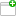This comment was attached:

arxcrash-19649-4274513080
#3
Comment posted byCrashBot
Jul 27, 15:50
A file was uploaded. cfg.iniThis comment was attached:

arxcrash-19649-4274513080
#4
Comment posted byCrashBot
Jul 27, 15:50
A file was uploaded. maps.txtThis comment was attached:

arxcrash-19649-4274513080
#5
Comment posted byCrashBot
Jul 27, 15:50
A file was uploaded. crash.txtThis comment was attached:

arxcrash-19649-4274513080